Abd Hamid, S. B., Chowdhury, Z. Z., and Karim, M. Z. (2014). "Catalytic extraction of microcrystalline cellulose (MCC) from Elaeis guineensis using central composite design (CCD)," BioRes. 9(4), 7403-7426.

#### Abstract

Cellulosic fiber extracted from a non-woody, monocotyledonous species of palm tree trunk (PTT) was hydrolyzed with different concentrations of FeCl3 in the presence of hydrochloric acid. The dissolution properties of the amorphous region of palm tree cellulosic fiber (PTC) can be enhanced in the presence of Fe3+ cations in acidic medium. The independent variables, including temperature (x1), time (x2), and concentration of metal chloride (FeCl3) catalyst (x3), were optimized using central composite design (CCD). Responses were measured in terms of percentage crystallinity (y1) and yield (y2) of the prepared hydrocellulose. Analysis of variance (ANOVA) showed that temperature was the most influential factor for hydrolyzing the amorphous sections of cellulose. Under optimum conditions, the percentage crystallinity (y1) and yield (y2) were 68.66% and 83.98%, respectively. Scanning electron microscopy (SEM) analysis and X-ray diffraction techniques were used to obtain more information about the surface morphology and crystallinity of the prepared microcrystalline cellulose (MCC). Infrared spectroscopy and thermal analysis were performed to observe the effect of hydrolysis on the finished products. It was concluded that the addition of FeCl3 salt in acid hydrolyzing medium can substantially increase the crystallinity of palm tree trunk cellulose with significant morphological changes to yield microcrystalline cellulose (MCC).

Catalytic Extraction of Microcrystalline Cellulose (MCC) from Elaeis guineensis using Central Composite Design (CCD)

Sharifah Bee Abd Hamid*, Zaira Zaman Chowdhury, and Md. Ziaul Karim

Cellulosic fiber extracted from a non-woody, monocotyledonous species of palm tree trunk (PTT) was hydrolyzed with different concentrations of FeCl3 in the presence of hydrochloric acid. The dissolution properties of the amorphous region of palm tree cellulosic fiber (PTC) can be enhanced in the presence of Fe3+ cations in acidic medium. The independent variables, including temperature (x1), time (x2), and concentration of metal chloride (FeCl3) catalyst (x3), were optimized using central composite design (CCD). Responses were measured in terms of percentage crystallinity (y1) and yield (y2) of the prepared hydrocellulose. Analysis of variance (ANOVA) showed that temperature was the most influential factor for hydrolyzing the amorphous sections of cellulose. Under optimum conditions, the percentage crystallinity (y1) and yield (y2) were 68.66% and 83.98%, respectively. Scanning electron microscopy (SEM) analysis and X-ray diffraction techniques were used to obtain more information about the surface morphology and crystallinity of the prepared microcrystalline cellulose (MCC). Infrared spectroscopy and thermal analysis were performed to observe the effect of hydrolysis on the finished products. It was concluded that the addition of FeCl3 salt in acid hydrolyzing medium can substantially increase the crystallinity of palm tree trunk cellulose with significant morphological changes to yield microcrystalline cellulose (MCC).

Keywords: Palm tree cellulose (PTC); Microcrystalline cellulose (MCC); Hydrocellulose; Central composite design (CCD); Percentage crystallinity; Amorphous sections

Contact information: Nanotechnology and Catalysis Center (NANOCAT), University Malaya, Kuala Lumpur 50603, Malaysia; *Corresponding author: sharifahbee@um.edu.my

INTRODUCTION

Recently, research has focused on obtaining sustainable, green, and ecofriendly materials for versatile applications (Abdul Khalil et al. 2012; Khalil et al. 2013). Cellulose, the main component of all biomass, is the most abundant bio-macromolecule found in nature. It has been estimated that more than 7.5 × 1010 tons of cellulose is produced annually (Habibi et al.2010). Irrespective of biomass sources, the long-chain cellulose polymer consists of a linear homo-polysaccharide composed of d-glucopyranose units linked together by 1-4 linkages. The repeating unit of cellulose is the dimer of glucose, known as cellubiose (Moon et al. 2011; Chowdhury et al. 2014). In addition to biodegradability and renewability, the fabrication of cellulosic materials into micro as well as nano dimensions enhances favorable characteristics such as excellent mechanical properties, high crystallinity, and low molecular weight (de Mesquita et al. 2010). Preparation of microcrystalline cellulose (MCC) derived from renewable sources is presently a hot investigation area. Microcrystalline cellulose, a porous, non-fibrous, white, odorless, purified, crystalline powder, is perhaps the best filler-binder used currently for pharmaceutical products (Ejikeme 2008; Li et al. 2013). It has been extracted by partial de-polymerization of native cellulose via hydrolysis with dilute mineral acids or enzymolysis (Ejikeme 2008). However, the acid extraction process is popular for industrial scale preparation of MCC due to its lower price and desirable properties of high crystallinity (Adel et al. 2011; Li et al. 2014).

The degree of crystallinity observed after hydrolysis of native cellulose is very important because it affects several properties, including compactibility, stabilizing capability for suspension, and water retention ability of MCC particles. Subsequently, this influences the flow ability of the MCC particles used as excipients or filler for final medicinal products (Azubuike et al. 2012). Because of its insoluble nature in common reagents like water, organic solvents, and dilute acids as well as its lubricating and hygroscopic properties, MCC has been widely used in cosmetics and the food industry as a fat replacement (Omray and Omray 1986; Uesu et al. 2000). Furthermore, it is used for pelletisation during the direct compression method as well as dry and wet formulation of capsules (Rowe et al. 2006). The chemical composition and morphological features of MCC significantly depends on the characteristics of the raw cellulosic materials and the extent and type of processing variables (Landin et al. 1993). Because cellulose is extracted from different origins such as soft wood, hard wood, or non-woody lignocellulosic residues and has diversified properties such as crystallinity, moisture content, surface area, porous structure, and molecular weight, it is expected that MCC procured from unlike sources would exhibit different properties. Basically, excipient materials like MCC for oral drugs are preselected depending on their compatibility and functionality to ensure the constancy and bioavailability of the finished pharmaceutical products; at the same time, the material used should have the prospect for industrial scale production. Thus, from an economic viewpoint, the use of biomass residues to extract cellulosic fiber and transforming them to MCC particles is advantageous.

Microcrystalline cellulose particles have previously been produced from hard wood pulp like Eucalyptus (Li et al. 2013), soft wood of Norway spruce (Bondeson et al. 2006), and cotton linters (Nada et al. 2009). However, some other non-woody biomass, such as soybean, corn stalk, oath, rice hulls, sugar beet pulp (Hanna et al. 2001; Adel et al. 2011), bagasse, maize cob (Okhamafe and Azubuike 1994; Okhamafe et al. 1995), wheat, barley, oath straw (Proenca 1999), groundnut shell, rice husks (Okhamafe et al. 1991), reed stalks (Saleh and El‐Ashmawy 1978), cereal straw (Jain et al. 1983), Indian bamboo (Ofoefule and Chukwu 1999), and Luffa cylindrica (Ohwoavworhua et al. 2004), have also been recognized as potential sources of MCC. During acid hydrolysis of cellulosic materials, amorphous regions are fragmented, resulting in a highly crystalline substrate (Costa et al. 2011). The amorphous regions are selectively hydrolyzed by strong mineral acids such as hydrochloric, nitric, and sulfuric acids (Follain et al. 2010; Hashaikeh and Abushammala 2011). However, to degrade the amorphous regions of cellulose while retaining the crystalline region almost unaltered to enhance the yield and crystallinity of MCC is challenging. Lack of process parameter optimization may result in char formation with drastic degradation of cellulosic backbone to yield other organic compounds as by-products rather than MCC particles. In this context, previous researchers have used different types of transition metal salts as catalysts such as FeCl3, CuCl2, and AlCl3 during acid hydrolysis of cellulose (Kamireddy et al. 2013). It was reported that a minute amount of FeSO4 can enhance the reducing sugar content during the hydrolysis of coniferous sawdust (Ren et al. 2012). Previous findings show that hydrolysis efficiency of cellulose can be upgraded significantly by the presence of Fe3+ and H+ ions simultaneously in the reaction medium (Sulaiman et al. 2012; Li et al. 2013).

Oil palm is considered to be a monocotyledonous, non-woody type of plant. The anatomical features of oil palm trunk differ chemically from hardwood and softwood species in terms of cellulose, hemicellulose, and lignin content (Akmar and Kennedy 2001; Nguyen and Tucker 2002; Dungani et al. 2013). The objective of this study was to hydrolyze the palm tree trunk cellulose (PTC) in the presence of FeCl3 catalyst during the acid hydrolysis process using response surface methodology (RSM). Central composite design (CCD) based on RSM is a combination of mathematical and statistical analysis of experimental observations. In the case of the conventional method, there is an amendment of only one parameter at a certain time interval while keeping the other parameters constant. Thus, a collective effect of all the parameters at a fixed time cannot be observed. This method is also time consuming and needs a lot of experiments for optimization. The RSM technique reduces process variability with accurate observation of output responses using a lesser number of experiments. This can establish an empirical relationship between the process variables with the desired responses or product characteristics. It delivers a complete experimental design for data assessment, model fitting, as well as a method for optimization (Chowdhury et al. 2011; 2012). Three individual factors of temperature, time, and concentration of metal ion catalyst were selected to investigate the hydrolysis mechanism of PTC in hydrochloric acid medium. The responses selected were percentage crystallinity of the hydrocellulose (MCC) and yield. Corresponding mathematical models were proposed. The effect of process variables on both the responses were analyzed in terms of analysis of variance (ANOVA).

EXPERIMENTAL

Materials

Catalytic hydrolysis of palm tree cellulose (PTC)

Palm tree cellulosic fiber (PTC) was supplied by the Malaysian Palm Oil Board (MPOB). It was procured from palm tree trunk biomass by the alkaline peroxide bleaching process (Mandal and Chakrabarty 2011). The dried and ground palm tree trunk was refluxed with 1 M sodium hydroxide solution for 8 h, where liquor to trunk ratio was 1:50 at room temperature. After alkali treatment, the residue contains 49.76% of cellulose, 8.5% hemicellulose, and 16.54% of lignin. It was bleached using 0.7% sodium chlorite solution (w/v) for 5 h. The reaction was carried out at pH 4 by adding buffer solution. After filtration, the delignified residue was washed with distilled water and boiled for 5 h with 5% sodium sulfite solution (w/v) to remove hemicellulose and lignin. The sample was further boiled with 17.5% sodium hydroxide solution for 5 h. The solution was filtered and the residue was washed and air dried; 50 mL of dimethylsulfoxide (DMSO) was added to the dried sample in a water bath for 3 h at 80 °C. Finally the product was filtered, washed with distilled water, and air-dried. The chemical composition of palm tree trunk, alkali- pretreated trunk, and the obtained bleached cellulosic sample, provided by MPOB, is enlisted in Table 1.

Iron (III) chloride hexahydrate and hydrochloric acid fuming 37% were of analytical grade and purchased from R & M Chemicals and Merck, respectively. The PTC was treated by varying the concentration of FeCl3 based on a basic design matrix (Table 2) whereby the solid liquid ratio was kept constant (1:30) in the presence of HCl (3 M). The cellulose sample with requisite amount of acid and catalyst were placed in a 100-mL round bottom flask and stirred on a magnetic hot plate. The flasks were heated to various temperatures and reaction times in a constant temperature oil bath. Samples were withdrawn from the reaction mixture under different reaction conditions preset by the experimental design (Tables 1 and 2).

Table 1. Chemical Composition of Palm Tree Trunk, Alkali-Pretreated Trunk, and Bleached Cellulosic Sample (PTC)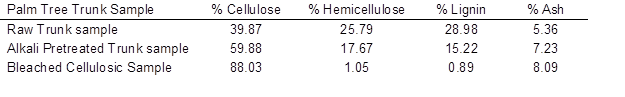The solution was centrifuged at 6000 rpm and washed with distilled deionized water until the filtrate was neutral. The sample was dried and the yield was calculated according to Eq. (1) (Li et al. 2013):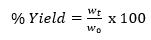(1)

Here, wt is the initial weight of PTC sample taken and w0 is the dry weight of powder finally obtained (Li et al. 2013).

Methods

Central composite design (CCD)

A two-level factorial design based on CCD using response surface methodology (RSM) was used to optimize the catalytic acid hydrolysis of PTC. This gives main and combined effects of variables on the responses under consideration. It also develops regression models with consequent optimization of the hydrolysis process. The influence of three independent variables, x1 (temperature), x2 (time), and x3 (concentration of FeCl3 catalyst), on two responses of percentage crystallinity (y1) and yield (y2), were evaluated. Twenty experimental runs were performed as suggested by the software. The variables and their range were selected based on preliminary studies. Independent variable ranges studied were: temperature (80 to 150 °C), time (2 to 4 h), and FeCl3 concentration (0.4 to 0.6 M), while HCl concentration was kept constant at 3 M for all of the experimental runs. Nevertheless, experimental runs were randomized to reduce the effects of residual errors. At the center point, six experiments were conducted under identical conditions to calculate repeatability of the data (Meyers and Montgomery 2002; Montgomery 2008). The complete design matrix depicting the levels of preferred variables is illustrated by Tables 2 and 3. The regression equation was developed based on the basic design matrix proposed by Tables 2 and 3.

Statistical analysis

Experimental data were fitted for regression analysis and to determine the regression coefficients. Statistical significance of the developed models was obtained by the analysis of variance (ANOVA) test. Process parameters were optimized to get a comprehensive region where the responses under consideration would be at a maximum. The performance of the response surface was investigated by using the regression polynomial equation. The generalized polynomial model proposed can be expressed as,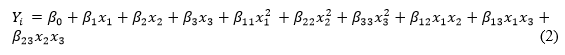where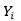is the desired response, β0 is the constant term, β1, β2, and β3 are the regression coefficients for linear effect terms, β11β22, and β33 are quadratic effects, and β12, β13, and β23are interaction effects. In this model, x1x2, and x3 are the process variables.

The ANOVA analysis of the experimental runs provided the regression coefficients for linear, quadratic, and interaction terms individually. The significance of each term for the responses was also evaluated by observing the F-ratio where the probability (p) is less than 0.05. The adequacy of the models was determined using model analysis, the lack-of-fit test, and coefficient of determination (R2) analysis. The experimental design matrix, data analysis, and optimization procedure were carried out using the software Design-Expert version 7.0.0 (Stat-Ease, Inc., USA)

Optimization process

The numerical optimization procedure was performed for percentage crystallinity (y1) and yield (y2). The software gave the optimal levels of three factors (x1, x2, and x3) to obtain the highest crystallinity percentage with maximum yield. The goal for each variable was kept within the studied range. Furthermore, a graphical technique in terms of contour plots with 3D response surfaces was used to visualize the relationship between the responses and experimental levels of each variable where one variable was kept constant at the center point and other two variables were varied within the experimental range.

Verification of developed models

The adequacy of the developed models for the responses was verified by conducting experiments under the optimum conditions suggested by the software. The experimental and predicted values of the responses were compared and percentage error was calculated in order to check the validity of predicted models.

Characterization

The surface morphology of the starting material (PTC) and obtained hydrolyzed PTC was checked by scanning electron microscopy (FEI Quanta 200F) under optimum conditions. The surface functional groups of native PTC and pretreated PTC were detected by Fourier transform infrared (FTIR) spectroscopy (FTIR-Bruker IFS 66/S; Germany). The spectra were recorded from 4000 to 400 cm-1. The crystalline structure of the samples was analyzed with a Cu-Kα radiation source by X-ray diffraction (XRD) (Bruker AXS D8 Advance). The percentage crystallinity was calculated according to Eq. (3) (Terinte et al. 2011),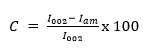(3)

where C is the percentage crystallinity, I002 is the maximum intensity of the 002 peak at 2θ = 22.50, and Iam is the intensity at 2θ = 18.70. Proximate analysis was carried out using thermal gravimetric analysis (TGA) equipment (Mettler Toledo TGA/SDTA 851e) to observe the weight loss of the native PTC and hydrolyzed PTC sample under optimum conditions.

RESULTS AND DISCUSSION

Development of Mathematical Model and Interpretation of Regression Analysis

The models depicting percentage crystallinity (y1) and yield (y2) for hydrolyzed palm tree cellulose (PTC) were developed based on the highest order polynomials. The additional terms are significant and the models were not aliased based on the sequential model sum of squares (Arami-Niya et al. 2011; Chowdhury et al. 2012). The parameters describing the reaction conditions in terms of actual and coded factors for model development were calculated from the basic design matrix of Table 2 and Table 3.

Table 2. Independent Variables for Acid Hydrolysis with their Actual and Coded Levels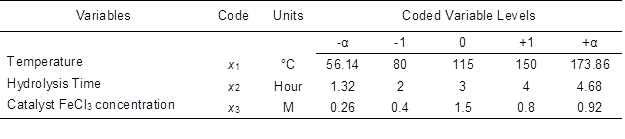The independent variables are coded as (-1, +1) interval where the low and high levels are represented by -1 and +1, respectively. The axial points are denoted as (0, 0, ±α), (0, ±α, 0), and (±α, 0, 0). Here, α indicates the distance between axial points with the center. The complete experimental matrix shown by Table 2 is composed of 6 axial points, 8 factorial points, and 6 center points where the experiments are conducted in identical conditions to determine the residual error.

For crystallinity percentage (y1) and yield (y2), quadratic models were developed. Equations (4) and (5) represent the final empirical equations expressed using the coded factors: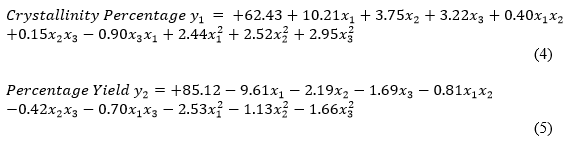The linear coefficients for the reaction variables of temperature (x1), time (x2), and catalyst concentration (x3) show the effect of that individual factor for hydrolysis of PTC. On the contrary, the coefficient represented by the product of two factors such as x1x2x2x3, and x3x1 illustrates the interaction effects on the responses. Second-order terms related to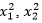and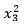represent a quadratic effect. A positive sign indicates a synergistic effect, whereas a negative sign indicates an antagonistic effect (Arami-Niya et al. 2011; Chowdhury et al. 2012).

Table 3. Experimental Design Matrix for Catalytic Acid Hydrolysis of Palm Tree Trunk Cellulosic Fiber (PTC)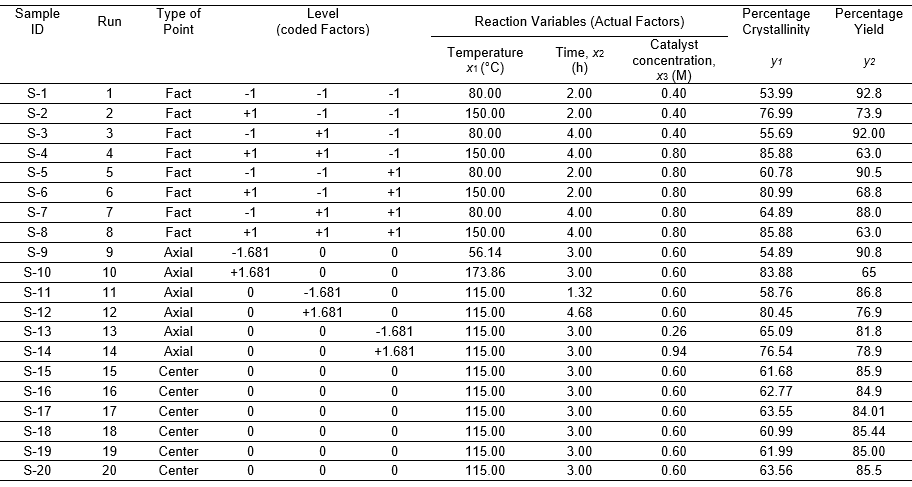Figures 1a and 1b show the linear plots of predicted versus experimental percentage crystallinity (y1) and yield (y2), respectively. As can be observed from these two plots, the predicted values for percentage crystallinity and MCC yield were fairly close to their experimental values. The R2 values for equations (4) and (5) were 0.95 and 0.96 for percentage crystallinity (Fig. 1a) and yield (Fig. 1b), respectively.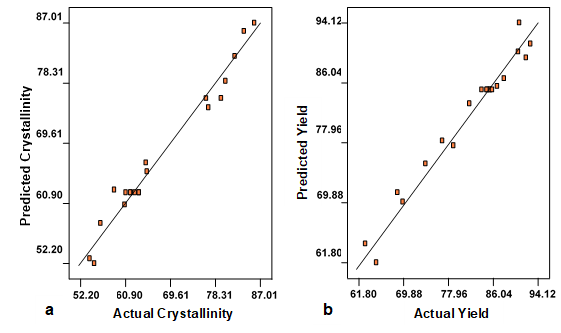Fig. 1. Predicted versus actual (a) percentage crystallinity y1 and (b) percentage yield y2of acid hydrolyzed palm tree cellulose (PTC)

Interpretation of Residual Plots

The normality of the data recorded for each experimental run can be checked by plotting the normal probability plot of the residuals for crystallinity percentage (y1) and yield (y2). The normal probability plot is a graphical representation for evaluating whether or not the data set obtained is approximately normally scattered. Basically, residual shows the difference between the experimental and the fitted or predicted value for model regression. If the data points on the plot are distributed fairly adjacent with the straight line, then it is assumed that the data are normally disseminated. From Fig. 2a and 2b, it is observed that the residual points were aligned with the straight line satisfactorily, signifying normal distribution.

Figures 3a and 3b show Studentized residuals versus the fitted value, whereas Figs. 4a and 4b show Studentized residuals versus experimental runs for percentage crystallinity (y1) and percentage yield (y2), respectively. The data points for residuals are randomly scattered about zero. This reveals that the variance of experimental observations is constant. All of the data points observed are found to fall within the range of +3 to -3. This ensures that no response transformation is necessary for the experimental design of this study.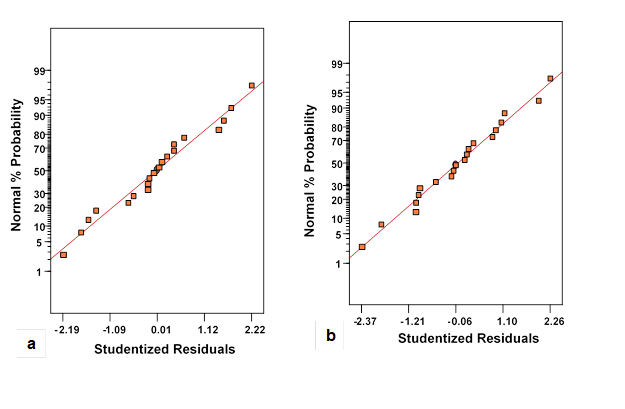Fig. 2. Normal probability plot residuals (a) percentage crystallinity (y1) and (b) percentage yield (y2) of acid hydrolyzed palm tree cellulose (PTC)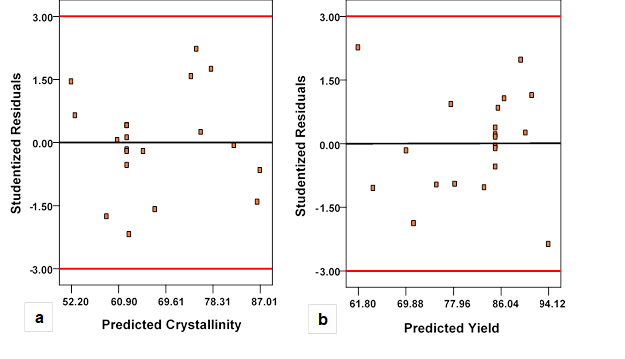Fig. 3. Studentized residuals versus fitted plot (a) percentage crystallinity (y1) and (b) percentage yield (y2) of acid hydrolyzed palm tree cellulose (PTC)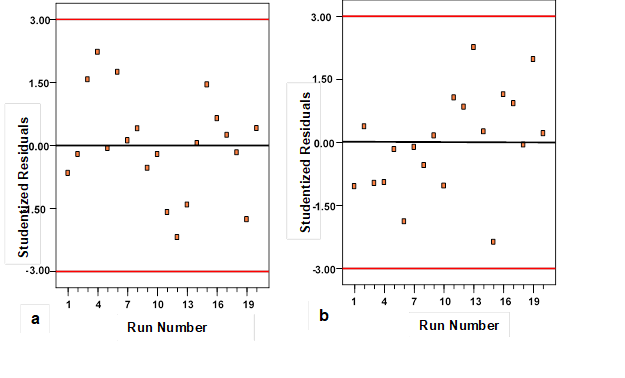Fig. 4. Studentized residuals versus run number (a) percentage crystallinity (y1) and (b) percentage yield (y2) of acid hydrolyzed palm tree cellulose (PTC)

The outlier t-plots for percentage crystallinity (y1) and yield (y2) are illustrated by Figs. 5a and 5b.

Outlier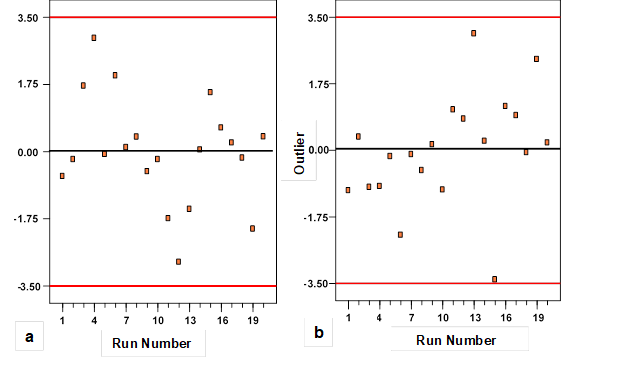Fig. 5. Outlier t plot for (a) percentage crystallinity (y1) and (b) percentage yield (y2) of acid hydrolyzed palm tree cellulose (PTC)

Data recording errors were analyzed by observing the t-plots. Nevertheless, outlier t-plots provide sufficient information about the region of independent variables where the fitted model has shown poor approximation to the true response surfaces (Chowdhury et al. 2012). It can be seen from Figs. 5a and 5b that most of the standard residuals fell within the interval range of ± 3.50. This further ensures that the data approximation for the developed models (Eqs. 1 and 2) to the response surface is fairly good exhibiting no data recording error.

ANOVA Analysis and Lack of Fit

The experimental results obtained are analyzed by analysis of variance (ANOVA) to measure the precision of the model. The statistical significance of the ratio of mean square variation and mean square residual error were verified using ANOVA. The regression coefficients of the models, corresponding R2 values, and lack of fit tests are provided in Table 4. The quality of the models can be further verified by the standard deviation. From Table 4, it was observed that experimental R2 values were in reasonable agreement with the adjusted R2. Moreover, the small values of co-efficient of variation (CV) as well as standard deviation reflect reproducibility of the model. The signal to noise ratio was determined in terms of adequate precision. For effective determination of the model, this value should be larger than 4. The adequate precision obtained for crystallinity (y1) and yield (y2) are 16.52 and 20.28, respectively. This further suggests that the developed models could be used to navigate the design.

Table 4. Statistical Parameters for ANOVA Analysis for Model Regression for Percentage Crystallinity (y1) and Yield (y2)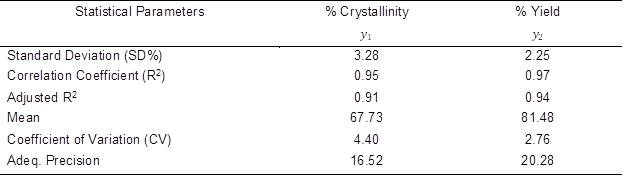The significance of linear, interaction, and quadratic model terms were determined using the F-test and p-value as presented in Tables 5 and 6. The most significant variable influencing the percentage crystallinity and yield was the linear term of temperature (x1). The results demonstrated that the regression models for the responses of yield and crystallinity percentage are significant by the F-test at the 5% confidence level.

The R2 values for these response variables were higher than 0.80 (0.95 to 0.97), which ensures satisfactory fitness of the regression models with the experimental data. In addition, the F-test was carried out to define the process that has a significant effect on both the responses. Generally, the alteration of the process parameter has a substantial effect on the quality characteristics when F is large. The results of ANOVA designated that the considered process parameters were highly significant factors affecting crystallinity percentage) and yield) of MCC. The value of probable F was less than 0.0001 for percentage crystallinity () and yield (), reflecting the significance of the model.

Table 5. ANOVA Analysis and Lack of Fit Test for Response Surface Model for Percentage Crystallinity (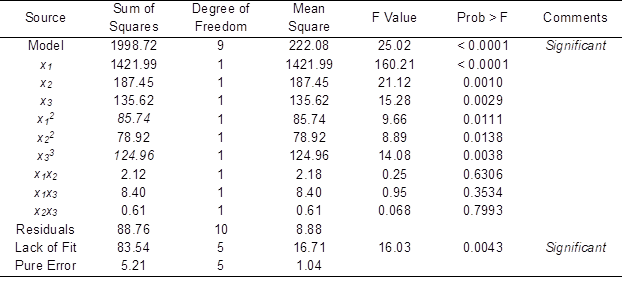Table 6. ANOVA Analysis and Lack of Fit Test for Response Surface Model for Percentage Yield ()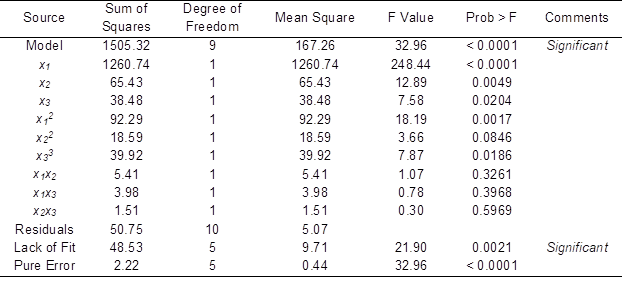From Table 5, it can be observed that temperature (x1), pretreatment time (x2), catalyst concentration (, and their quadratic terms of (), (), and were significant model terms. Referring to Table 6 for the quadratic model of MCC yield, x1, x2, and , as well as the quadratic terms of and were significant model terms, whereas other interaction terms are negligible relative to the response. For percentage crystallinity (y1) and yield (y2), temperature had the greatest effect on these responses by showing the highest F values of 1421.99 and 1260.74, respectively, as shown in Tables 5 and 6. Hydrolyzing time ( and catalyst concentration ( had moderate effects, whereas the interaction effect of temperature and time (x1x2) and temperature and catalyst concentration (x1x3) were more prominent than the other two interaction terms relative to the percentage crystallinity and yield, respectively.

Process Variables Optimization

When producing microcrystalline cellulose (MCC), relatively high product yields are expected so that further degradation of the crystalline portion of MCC in harsher conditions can be prevented. The most important property of microcrystalline cellulose is its percentage crystallinity. Therefore, in a practical manufacturing process, a compromise should be made between the yield and the percentage crystallinity to ensure selective acid hydrolysis of the amorphous region, as well as to prevent char formation by using comparatively mild conditions. However, optimization of both of these responses under the same condition is difficult because the interest region of the factors is different. As observed from the basic design matrix, when the percentage crystallinity increases, the yield decreases and vice versa. Therefore, in order to achieve a compromise between these two responses, the numerical optimization menu is selected. In order to optimize the preparation conditions, the targeted criteria is set as maximum values for the two responses, while the values of the three variables (temperature, time, and catalyst concentration) are set in the ranges being studied. The sample has been prepared under optimum conditions and the experimental results are compared with the predicted values. The percentage deviation between the predicted and experimental conditions is evaluated and presented in Table 7.

Table 7. Process Parameter Optimization for Acid Hydrolysis of MCC in Presence of FeCl3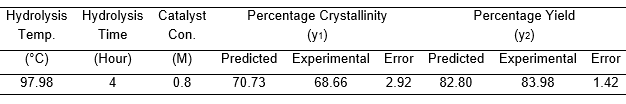Under identical conditions of temperature, time, and acid concentration, the reaction was conducted without adding FeCl3 catalyst. The prepared sample exhibited a crystallinity index of 58.22%.

Interpretation of Surface Contour Plots

Figure 6a is constructed to show the three-dimensional response surfaces with a contour plot, which shows the combined effect of two significant variables (temperature and time) on percentage crystallinity ) of extracted microcrystalline cellulose (MCC) where the catalyst concentration is kept at the center point, which is the zero level (0.60). Figure 6b shows the combined effect of hydrolysis temperature and catalyst concentration on percentage crystallinity, where the hydrolyzing time is fixed at the zero level, which is 3 h. In this work, the three variables studied were found to have synergistic effects on percentage crystallinity. Percentage crystallinity was increased significantly when temperature and time were at the maximum (Fig. 6a). This is expected, as the progressive increase of temperature and hydrolyzing time increases the diffusion of hydrochloric acid as well as the catalyst solution inside the cellulosic matrix which would initiate the disintegration of the amorphous region of cellulose. Sufficient contact time also causes physical swelling of PTC, which in turn increases the surface area of the sample. Consequently, hydrolysis efficiency becomes higher. However, prolonged contact time for hydrolyzing is not suggested, as it might decrease the yield with a successive decrease of crystallinity.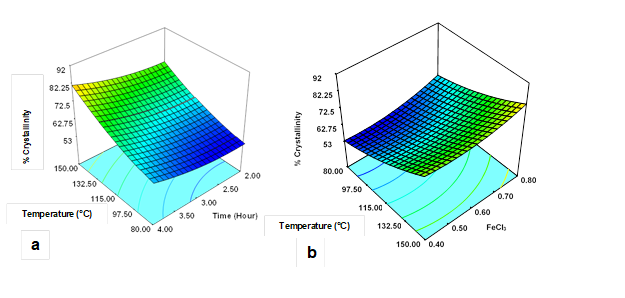Fig. 6. Response surface and contour plots of the combined effects of (a) temperature and time and (b) temperature and catalyst concentration on percentage crystallinity (y1) of acid hydrolyzed MCC when the other two variables are at center points

Increasing the concentration of the metal ion catalyst, which was acting as a Lewis acid, increased the percentage crystallinity (. At the initial stage, Fe3+ forms a coordination complex with water to yield H3O+ ions. Fe3+ will form a coordinate covalent bond with 6 water molecules resulting in metal-ligand complexes of [M (H2O)n]Z+, where M represents the cation, Z is the oxidation state of the cation, and n is the solvation number, which is between 4 and 6. The reaction scheme is shown by following equations (Li et al. 2013):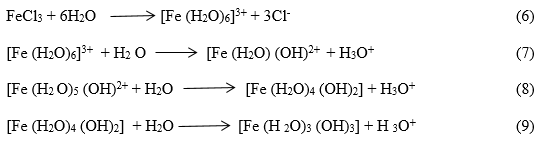The central metal ions polarize the water molecules by withdrawing electron density. This makes the hydrogen atoms in O-H bond more electropositive. The complex ions deprotonate, making the solution acidic. Enhanced acidity due to presence of H3O+ ions breaks down the glycosidic linkage between the glucose units of cellulose. Oxygen atoms of the glucose unit in cellulose can readily form intermediate complexes by absorbing Fe3+ ions. This oxygen-iron co-ordination complex increases pyranose bond length and bond angle, resulting in lower activation energy of the reaction. Thus hydrolysis efficiency is greater (Zhao et al. 2011). However, as stated earlier, process parameters must be optimized to reduce the formation of char with the production of furfural and 5-hydroxymethylfurfural as secondary products according to the following reaction scheme: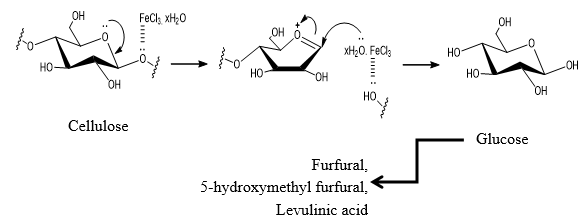Fig. 7. Proposed reaction scheme for catalytic acid hydrolysis of palm tree cellulose (PTC)

Figs 8a and 8b show the 3D response surfaces that are constructed to reveal the effects of reaction variables on MCC yield. Figure 8a represents the combined effect of temperature and time on the response, where the catalyst concentration is fixed at the zero level (0.6 M). Figure 8b illustrates the effect of temperature and catalyst concentration on the same response, where time is fixed at the zero leve1 (3 h).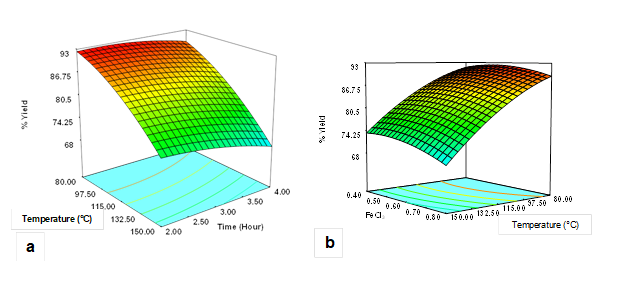Fig. 8. Response surface and contour plots of the combined effects of (a) temperature and time and (b) temperature and catalyst concentration on percentage yield (y2) of acid hydrolyzed MCC when the other two variables are at center points

In general, yield was found to decrease with increased temperature, time, and catalyst concentration. As can be seen from both the plots (Figs. 8a and 8b), temperature was more dominant relative to the yield as compared to the other two variables. The lowest yield was obtained when temperature was at the maximum point (173.86 °C, Sample 10) within the studied range as depicted by the design matrix of Table 2. This is due to chemical degradation of cellulose in acid hydrolyzing medium (Zhao et al. 2011).

Physiochemical Characterization of the Hydrocellulose

The changes in the structures of the treated MCC particles due to acid hydrolysis in the presence of FeCl3 catalyst were confirmed by the images obtained from scanning electron microscopy (Fig. 9). The morphological changes in the structures of the hydrolyzed palm tree cellulose (PTC) compared to the native cellulose fibers were confirmed by the images obtained from scanning electron microscopy (Fig. 9). The results confirmed obvious size reduction and fractures of the PTC sample treated under optimum conditions.

Figure 9a illustrates the structure of native PTC, which is comparatively uniform, flat, and smooth even at lower magnification. This exhibits the rigid and well-ordered structure of cellulose. At higher magnification (Fig. 9b), some folds were observed over the surface of PTC. This type of structural feature of native palm tree cellulose provides adequate contact area for accessibility of FeCl3 catalyst as well as HCl acid itself (Li et al. 2013).

Figure 9c shows images of the sample treated under optimum conditions where the HCl concentration was fixed at 3 M, the catalyst concentration at 0.8 M, hydrolyzing time of 4 h, and hydrolyzing temperature of 97.98 °C. The fibers were degraded into fragments due to chemical swelling and acid hydrolysis. The results are in agreement for acid hydrolysis of waste banana peel fibers (Elanthikkal et al. 2010). The end products are agglomerated, which are similar to the MCC particles derived from Norway spruce previously observed by Bondsen et al. (2006). Loss of orderly fibrillar structure of treated PTC indicates surface etching coupled with a certain degree of erosion. Comparatively large number of cracks and crevices are visible over the surface of the hydrolyzed PTC (Fig. 9d).

These cracks appeared due to quick diffusion of acid and metal ions inside the cell wall of the fiber. The dense structure visible in Fig. 9d represents the high crystalline region of cellulose. Similar morphological features are reported for catalytic acid hydrolysis of sulfate pulp extracted from hard wood of Eucalyptus using FeCl3 as the catalyst (Li et al. 2013; 2014).

X-ray diffraction

The XRD patterns of the native and hydrolyzed PTC fiber are illustrated in Fig. 10. It was observed previously that the major diffraction peak for cellulose can be observed for 2θ ranging between 22° and 23° as a primary peak, whereas a secondary peak is in the range of 16° to 18° (Li et al. 2014).